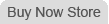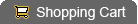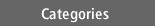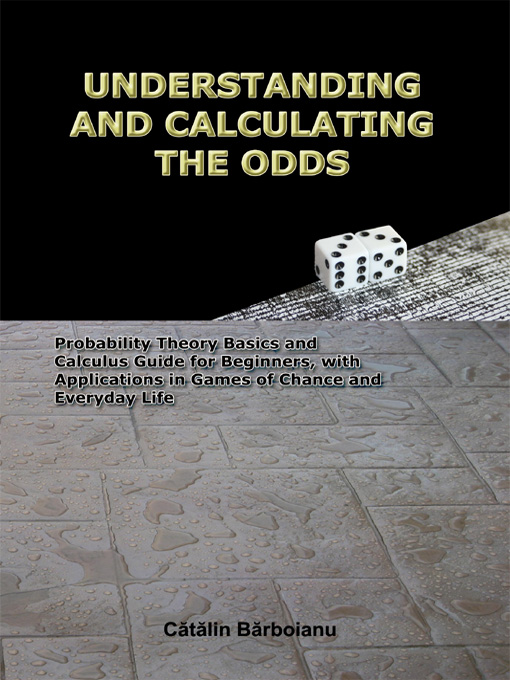Understanding and Calculating the Odds: Probability Theory Basics and Calculus Guide for Beginners, with Applications in Games of Chance and Everyday Life
Condition: New
Price:  \$18.00Description:

Author: Catalin Barboianu

• Paperback: 300 pages
• Publisher: INFAROM (May 21, 2006)
• Language: English
• ISBN-10: 9738752019
• ISBN-13: 978-9738752016
• Product Dimensions: 9 x 6 x 0.6 inches
• List price: \$29 (our price: \$18, you save \$11)
• Man's daily life is full of decisional situations. Whether we have math skills or not, we frequently estimate and compare probabilities, sometimes without realizing it, especially when making decisions. But probabilities are not just simple numbers attached objectively or subjectively to events, as they perhaps look, and their calculus and usage is highly predisposed to qualitative or quantitative errors in the absence of proper knowledge. That is why a book explaining the probability concept and its interpretations and applications for non-mathematicians is a necessity. This is an enlightening journey through the world of probability theory. Its multiple goals are to help the reader understand what probability really means, to teach the reader how to rigorously perform and apply the probability calculus, even without a solid mathematical background, and to stimulate the reader to go deeper into the notions involved. In the first part, the author tries to build a clear image of the probability concept by reconstructing its mathematical definition step by step through its constituent notions. It starts with a general presentation of the conceptual ensemble word - definition - notion - model any theory is based on when trying to reproduce reality. Then, the probability notion is defined and explained starting from the classical definition to the definition for the countable case; then probability is presented as a limit and as a measure. This book presents not only the mathematical concept of probability, but also its philosophical aspects, the relativity of probability and its applications and even the psychology of probability. All explanations are made in a comprehensible manner and are supported with suggestive examples from nature and daily life and even with challenging math paradoxes. After these points are laid out the math chapter follows. It contains all the notions and principal theoretical results that ground Probability Theory, starting with fundamental notions like Sets, Functions, Boole algebras, and Sequences, and continuing with Measure Theory Basics - Tribes, Borel sets, Measurable spaces, and Measure, ending with Field of events, Sigma-fields, Probability, Conditional probability, Discrete random variables, Classical probability distributions, and Convergence. And, of course, it includes all important theorems and results dealing with them. A special section is dedicated to Combinatorics and combinatorial calculus. Readers with no minimal mathematical background may choose to skip this chapter because the teaching material is structured for developing probability calculus skills based on algorithmic procedures. This is the subject of the chapter titled Beginners' Calculus Guide, in which the reader is taught to apply the properties of probability and to perform calculations in practical applications. The skills acquired here can be practiced on the more than 200 solved and unsolved problems and exercises in the book. Everyone should find something of interest here: philosophers and mathematicians may focus on the sections on philosophical matters of the probability model and decisional matters, students and non-mathematicians can find solid A to Z teaching material about Probability Theory and the practical person can find all the tools needed to apply and perform probability calculus without a teacher.

Return Policy:I do not accept returns
Shipping:

# Destination: United States

STANDARDStandard\$6.00\$1.00

# Destination: Worldwide

STANDARDStandard\$12.00\$3.00

# Destination: United States & United Kingdom & Australia

Price:  \$18.00